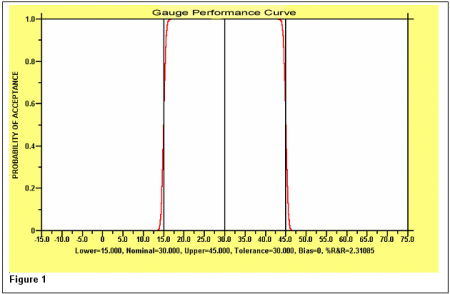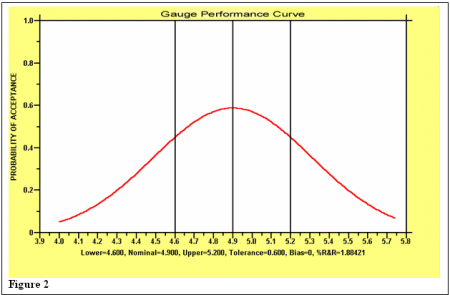## MSA: Measuring your measurement system with a performance curve

By Jackie Graham, Ph.D.

As measurement system variation increases, the probability of getting a correct value from a measurement system reduces. This concept is portrayed best by using a ‘gage performance curve.’ The example ‘gage performance curve’ shown in figure 1, demonstrates an excellent measurement system where the measurement variation is low, with an R&R (reproducibility and repeatability) of less than 10%.Now, to explain what the chart means and how you interpret it. The x-axis shows the range of actual values of the product being measured. The y-axis shows the probability of accepting a product of any of the values using the current measurement system. The straight line in the center of the chart is the center of the specification, while the two straight lines either side of the center line represent the tolerance range. The curve represents the probability of accepting product to the tolerance. From the chart, it can be seen that if the true product value is 30 (the center of the specification) the chance of its being accepted using the measurement system studied is 1.0 or 100%, as it should be. If the product value is 10, well below the lower specification, the chance of its being accepted is 0 or 0%, again as it should be, since it is well below specification. Although a value of 14 is below the lower specification (15), the curve shows it has a small chance of being accepted in error. The curve shows that a product with a value of 16 has a high chance of being accepted, ideally this would be 100%, but due to the variation in the measurement system it has a slight chance of being rejected.

Ideally, the ‘gage performance curve’ should show a reading of 0% up to the lower specification, go straight to 100%, and remain at 100% to the upper specification, then go back to 0%. However, no measurement system is perfect; there will always be some chance of accepting or rejecting product in error, graphically depicted by the gage performance curve.

A measurement system with extremely high variation, say with an R&R of 300% of the tolerance, (generally the maximum acceptable R&R is 30%) would show a very different ‘gage performance curve.’ Figure 2 depicts such a curve.This chart graphically demonstrates the impact of a highly variable measurement system. In this example, if the product has a value in the center of the specification it only has about a 60% chance of being accepted. This means that when the reading is taken from the measuring equipment, it has a 60% chance of producing a result inside the specification, and a 40% chance of producing a result outside the specification. As the curve shows, product that is way outside the specification still has a chance of being accepted. In this case, the specification is 4.6 to 5.2, yet product could be accepted from less than 4.00 to more than 5.75. This is quite a range when compared to the specification range of 0.6! If you think this kind of measurement system does not exist, unfortunately you are wrong. They occur all too frequently!

When setting up a measurement system it is essential to ensure that it is adequate for its purpose. The only way this can be assured is by completing an R&R study. If this is not completed, expect to accept product that is out-of-specification, and to reject acceptable product in error. The costs of poor measurement systems are enormous.

So, how do your measurement systems measure up? GAGEpack allows easy assessment of measurement systems and subsequent analysis using tools like the gage performance curve. Download a free trial now.